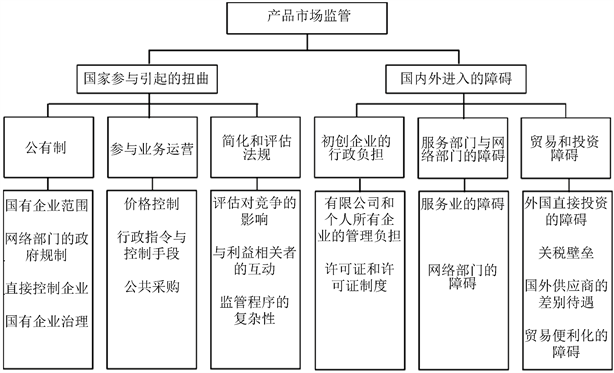#### 期刊菜单

Research on the Trade and Investment Effects of State-Owned Enterprises and Competitive Neutrality
DOI: 10.12677/WER.2019.84008, PDF, HTML, XML, 下载: 536  浏览: 1,243  国家社会科学基金支持

Abstract: The practice of “competitive neutrality” of state-owned enterprises in regional trade and invest-ment agreements of developed countries, and privatization of countries since the 1990s, has led to a decline in trade and investment barriers related to state-owned enterprises and competitive neutrality. This article attempts to use the gravity model, according to the national economic policy system indicators published by the OECD, and the publicly owned indicators under the government control factors of the state-owned enterprises are selected as independent variables. The 35 OECD countries involved and 40 countries from Brazil, China, India, Russia, and South Africa were selected as sample countries to study the impact of the regulation of trade and investment barriers on state-owned enterprises on bilateral trade and investment effects. Empirical evidence shows that the reduction of barriers to state-owned enterprises can effectively promote the development of bilateral trade and investment.

1. 引言

2. 国有企业及其竞争中立的宏观度量Figure 1. Product market regulatory indicatorsTable 1. Composition of public ownership index

3. 贸易效应实证研究

3.1. 选取模型

$\begin{array}{l}{\text{lnIMPORT}}_{ijt}={\beta }_{0}+{\beta }_{1}\mathrm{ln}{\text{GDP}}_{it}+{\beta }_{2}\mathrm{ln}{\text{GDP}}_{jt}+{\beta }_{3}\mathrm{ln}DISTANC{E}_{ij}+{\beta }_{4}\mathrm{ln}{\text{POP}}_{it}\\ \text{\hspace{0.17em}}\text{\hspace{0.17em}}\text{\hspace{0.17em}}\text{\hspace{0.17em}}\text{\hspace{0.17em}}\text{\hspace{0.17em}}\text{\hspace{0.17em}}\text{\hspace{0.17em}}\text{\hspace{0.17em}}\text{\hspace{0.17em}}\text{\hspace{0.17em}}\text{\hspace{0.17em}}\text{\hspace{0.17em}}\text{\hspace{0.17em}}\text{\hspace{0.17em}}\text{\hspace{0.17em}}\text{\hspace{0.17em}}\text{\hspace{0.17em}}\text{\hspace{0.17em}}\text{\hspace{0.17em}}+{\beta }_{5}\mathrm{ln}{\text{POP}}_{jt}+{\beta }_{6}\mathrm{ln}SO{E}_{it}+{\beta }_{7}LANGUAG{E}_{ij}+{\beta }_{8}ADJACEN{T}_{ij}+{\beta }_{9}FT{A}_{ij}+{\epsilon }_{ijt}\end{array}$, (1)

$\begin{array}{l}{\text{lnEXPORT}}_{ijt}={\beta }_{0}+{\beta }_{1}\mathrm{ln}{\text{GDP}}_{it}+{\beta }_{2}\mathrm{ln}{\text{GDP}}_{jt}+{\beta }_{3}\mathrm{ln}DISTANC{E}_{ij}+{\beta }_{4}\mathrm{ln}{\text{POP}}_{it}\\ \text{\hspace{0.17em}}\text{\hspace{0.17em}}\text{\hspace{0.17em}}\text{\hspace{0.17em}}\text{\hspace{0.17em}}\text{\hspace{0.17em}}\text{\hspace{0.17em}}\text{\hspace{0.17em}}\text{\hspace{0.17em}}\text{\hspace{0.17em}}\text{\hspace{0.17em}}\text{\hspace{0.17em}}\text{\hspace{0.17em}}\text{\hspace{0.17em}}\text{\hspace{0.17em}}\text{\hspace{0.17em}}\text{\hspace{0.17em}}\text{\hspace{0.17em}}\text{\hspace{0.17em}}\text{\hspace{0.17em}}+{\beta }_{5}\mathrm{ln}{\text{POP}}_{jt}+{\beta }_{6}\mathrm{ln}SO{E}_{it}+{\beta }_{7}LANGUAG{E}_{ij}+{\beta }_{8}ADJACEN{T}_{ij}+{\beta }_{9}FT{A}_{ij}+{\epsilon }_{ijt}\end{array}$, (2)

$\begin{array}{l}{\text{lnTRADE}}_{ijt}={\beta }_{0}+{\beta }_{1}\mathrm{ln}{\text{GDP}}_{it}+{\beta }_{2}\mathrm{ln}{\text{GDP}}_{jt}+{\beta }_{3}\mathrm{ln}DISTANC{E}_{ij}+{\beta }_{4}\mathrm{ln}{\text{POP}}_{it}\\ \text{\hspace{0.17em}}\text{\hspace{0.17em}}\text{\hspace{0.17em}}\text{\hspace{0.17em}}\text{\hspace{0.17em}}\text{\hspace{0.17em}}\text{\hspace{0.17em}}\text{\hspace{0.17em}}\text{\hspace{0.17em}}\text{\hspace{0.17em}}\text{\hspace{0.17em}}\text{\hspace{0.17em}}\text{\hspace{0.17em}}\text{\hspace{0.17em}}\text{\hspace{0.17em}}\text{\hspace{0.17em}}\text{\hspace{0.17em}}\text{\hspace{0.17em}}+{\beta }_{5}\mathrm{ln}{\text{POP}}_{jt}+{\beta }_{6}\mathrm{ln}SO{E}_{it}+{\beta }_{7}LANGUAG{E}_{ij}+{\beta }_{8}ADJACEN{T}_{ij}+{\beta }_{9}FT{A}_{ij}+{\epsilon }_{ijt}\end{array}$, (3)

3.2. 样本及数据来源

3.3. 回归结果与分析

(1)~(3)均经过对数处理，因此能够消除一定的异方差性。其次，在回归之前，先利用Eviews8.0对(1)~(3)的初始模型进行平稳性检验。检验结果如表2所示。三个方程结果均通过了显著性为1%的平稳性检验，所以三个方程中的因变量均为平稳时间序列。Table 2. P value of stationary testTable 3. Results of trade gravity model equation regression

(1)的回归结果与理论假设一致，经济规模(GDP)、人口、语言、双方签署FTA和陆地接壤对贸易有明显的正向促进作用，而距离、国有企业因素在一定程度上抑制了双边贸易。因此，引力模型实证表明国有企业因素壁垒的削减能有效促进双边贸易的发展。

4. 投资效应实证研究

4.1. 选取模型

Santos Silva和Teneyro (2006)提出泊松伪最大似然(PPML)估计是解决引力模型异方差和零值因变量有偏估计问题的最优选择，他们使用蒙特卡罗模拟法，将PPML与使用对数线性化模型的最小二乘法(OLS)的估计结果进行比较，发现在存在异方差性的情况下，使用对数线性化模型获得的估计结果严重偏差，而泊松伪最大似然(PPML)的结果均保持稳健，证明了泊松伪最大似然(PPML)估计器在估计引力模型方面的性能优于传统的线性估计 。

$\begin{array}{l}\mathrm{ln}{\text{FDI}}_{\text{INWARD}}{}_{ijt}={\beta }_{0}+{\beta }_{1}\mathrm{ln}{\text{GDP}}_{it}+{\beta }_{2}\mathrm{ln}{\text{GDP}}_{jt}+{\beta }_{3}\mathrm{ln}{\text{GDP}}_{\text{GROW}}{}_{it}+{\beta }_{4}\mathrm{ln}SO{E}_{it}\\ \text{\hspace{0.17em}}\text{\hspace{0.17em}}\text{\hspace{0.17em}}\text{\hspace{0.17em}}\text{\hspace{0.17em}}\text{\hspace{0.17em}}\text{\hspace{0.17em}}\text{\hspace{0.17em}}\text{\hspace{0.17em}}\text{\hspace{0.17em}}\text{\hspace{0.17em}}\text{\hspace{0.17em}}\text{\hspace{0.17em}}\text{\hspace{0.17em}}\text{\hspace{0.17em}}\text{\hspace{0.17em}}\text{\hspace{0.17em}}\text{\hspace{0.17em}}\text{\hspace{0.17em}}\text{\hspace{0.17em}}\text{\hspace{0.17em}}+{\beta }_{5}\mathrm{ln}DISTANC{E}_{ij}+{\beta }_{6}ADJACEN{T}_{ij}+{\beta }_{7}FT{A}_{ij}+{\beta }_{8}LANGUAG{E}_{ij}\\ \text{\hspace{0.17em}}\text{\hspace{0.17em}}\text{\hspace{0.17em}}\text{\hspace{0.17em}}\text{\hspace{0.17em}}\text{\hspace{0.17em}}\text{\hspace{0.17em}}\text{\hspace{0.17em}}\text{\hspace{0.17em}}\text{\hspace{0.17em}}\text{\hspace{0.17em}}\text{\hspace{0.17em}}\text{\hspace{0.17em}}\text{\hspace{0.17em}}\text{\hspace{0.17em}}\text{\hspace{0.17em}}\text{\hspace{0.17em}}\text{\hspace{0.17em}}\text{\hspace{0.17em}}\text{\hspace{0.17em}}\text{\hspace{0.17em}}+{\beta }_{9}\mathrm{ln}{\text{TRADEOPEN}}_{it}+{\beta }_{10}\mathrm{ln}FDIRESTRICTION{S}_{ij}+{\epsilon }_{ijt}\end{array}$, (4)

4.2. 样本及数据来源

4.3. 回归结果与分析

(4)的回归结果中，国有企业因素( ${\text{SOE}}_{it}$ )通过了显著性水平为5%的t检验，贸易限制指数( ${\text{FDIRESTRICTIONS}}_{it}$ )未通过显著性检验，其他自变量均通过了显著性水平为1%的z检验。剔除贸易限制指数( ${\text{FDIRESTRICTIONS}}_{it}$ )后，得到(4)’，对(4)’进行回归，国有企业因素( ${\text{SOE}}_{it}$ )通过了显著性水平为5%的z检验，其他自变量均通过了显著性水平为1%的z检验。Table 4. Results of FDI gravity model equation regression

5. 结论

NOTES

12018年指标期刊正在发表中，期刊信息为Vitale, C., et al. (2019). “2018 Update of the OECD PMR Indicators and Database - Policy Insights for OECD Countries”, OECD Economics Department Working Papers No. 资料来源参见 http://www.oecd.org/economy/reform/indicators-of-product-market-regulation/。

22018年的PMR指标在结构上有调整，2015年PMR是由政府控制、企业障碍、贸易和投资障碍三项指标构成，2018年国家参与引起的扭曲和国内外进入障碍两项指标构成，国家参与引起的扭曲和国内外进入障碍两项指标分别对应于2015年的政府控制和投资障碍两项指标，而2015年的企业障碍的子项目被整合至国家参与引起的扭曲和国内外进入障碍两项指标中。但是，公有制项下的国有企业范围、网络部门的政府规制、直接控制企业和国有企业治理四项子项目维持不变。

3根据OECD市场监管指标数据库整理。

http://www.oecd.org/economy/reform/indicators-of-product-market-regulation/。

  李宇英. “竞争中立”规制水平的国际比较研究[J]. 复旦学报(社会科学版), 2019, 61(2): 172-182.  Koske, I., et al. (2015) The 2013 Update of the OECD’s Database on Product Market Regulation: Policy Insights for OECD and Non-OECD Countries. OECD Economics Department Working Papers, No. 1200, OECD Publishing, Paris, p. 10, 57.  Santos Silva, J.M.C. and Tenreyro, S. (2006) The Log of Gravity. The Review of Economics and Statistics, 88, 641-658. https://doi.org//10.1162/rest.88.4.641  Mistura, F. and Roulet, C. (2019) The Determinants of Foreign Direct In-vestment: Dostatutory Restrictions Matter? OECD Working Papers on International Investment, 2019/01, OECD Publishing, Paris, 18-35.